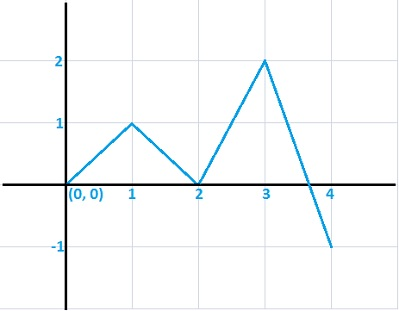# Designing a function. Sketch the graph of a continuous function f on [0,4] satisfying the given...

## Question:

Designing a function. Sketch the graph of a continuous function f on {eq}[0,4] {/eq} satisfying the given properties. f has a local max at x = 1, f has a local min at x = 2, f has an absolute max at x = 3, and f has an absolute min at x = 4.

## Sketching a Graph:

A graph is one of the best ways to show the properties of a function like the local maximum or minimum values on a specific interval or global maximum or minimum values on an interval.

## Answer and Explanation:Explanation:

The graph is unbroken on the interval {eq}[0,\,4] {/eq}, hence it is continuous on {eq}[0,\,4] {/eq}.

In the...

See full answer below.

Become a Study.com member to unlock this answer! Create your account